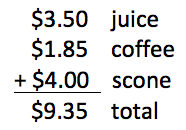### Learning Objectives

• Use addition and subtraction when evaluating expressions with decimals

Understanding how to work with decimals when making calculations involving money is very important in business. We know that $1$ is the same as $1.00$. But the way we write $1\text{, or }1.00$, depends on the context. In the same way, integers can be written as decimals with as many zeros as needed to the right of the decimal.

$\begin{array}{cccc}5=5.0\hfill & & & -2=-2.0\hfill \\ 5=5.00\hfill & & & -2=-2.00\hfill \\ 5=5.000\hfill & & & -2=-2.000\hfill \end{array}$
and so on $\dots$

So when making calculations with money, you may have to fill in zeros as place holders to correctly align the values.

Let’s say you stopped by Bouchon Bakery for breakfast this morning: the orange juice was three and a half dollars, the coffee was $1.85, and the scone was$4. All three items first need to be represented in dollars in cents so we can correctly add the numbers.We lined up the dollars under the dollars and the cents under the cents, with the decimal points lined up between them. Then we just added each column, as if we were adding whole numbers. By lining up decimals this way, we can add or subtract the corresponding place values just as we did with whole numbers.

1. Write the numbers vertically so the decimal points line up.
2. Use zeros as place holders, as needed.
3. Add or subtract the numbers as if they were whole numbers. Then place the decimal in the answer under the decimal points in the given numbers.

We’ll practice adding decimals in the example below. Remember — just like with whole number addition — you might have to “carry” a ten to the next place value as you add each column.

### example

Add: $3.7+12.4$

Solution

 $3.7+12.4$ Write the numbers vertically so the decimal points line up. $\begin{array}{c}\hfill 3.7\\ \hfill \underset{\text{_____}}{+12.4}\end{array}$ Place holders are not needed since both numbers have the same number of decimal places. Add the numbers as if they were whole numbers. Then place the decimal in the answer under the decimal points in the given numbers. $\begin{array}{c}\hfill \stackrel{1}{3}.7\\ \hfill \underset{\text{_____}}{+12.4}\\ \hfill 16.1\end{array}$

### example

Add: $23.5+41.38$

### try it

In the following video we show another example of how to add decimals.

Subtracting decimals follows the same process as adding decimals. Remember — just like with whole number subtraction — you may have to “borrow” ten from the next place value as you subtract each column.

### example

How much change would you get if you handed the cashier a $20$ bill for a $14.65$ purchase? We will show the steps to calculate this in the next example.

Subtract: $20 - 14.65$

### example

Subtract: $2.51 - 7.4$

### try it

This video shows another example of how to subtract one decimal from another.

## Contribute!

Did you have an idea for improving this content? We’d love your input.